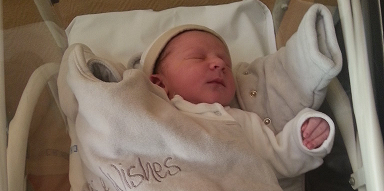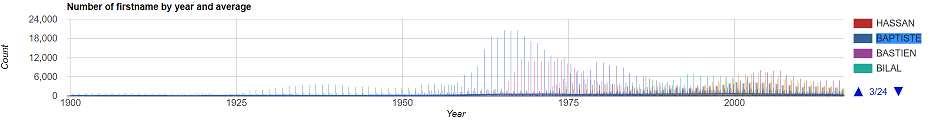11 May 2018

# Finding your baby name with fsharpFirst of all I am glad to have another child in my familly.

He is my 2nd boy, so it was really hard for us to find another boy name.

I found this french data : https://www.insee.fr/fr/statistiques/fichier/2540004/nat2016_txt.zip but there is 14596 boys names; the choice is hard!

How to find the pretty well name for my little boy ?

## The Problem

I was using this site : https://dataaddict.fr/prenoms/ and I saw one problem : Lack of classification.

In spite of that point, the idea is good : to see how and when a name is used with stats.

• I would like to see names by their properties (long, short, composed, …).
• And group them phonetically
• See less than 100 names in a graph of usage per year

So the aim of this hack was to provide a way to reduce the amount of name that we don’t wan’t due to their caracteristics.

## Parsing the data

FSharp has a fast data analytics in its core so it is just one line to parse the csv.

``````#r "packages/FSharp.Data/lib/net45/FSharp.Data.dll"

open FSharp.Data

type FirstNameStats = CsvProvider< "nat2016_txt/nat2016.txt", Separators = "\t", HasHeaders=true >

``````

Now build a function that returns all name for a given gender by year.

``````module Int32 =
let tryInt x =
match System.Int32.TryParse(x) with
| true, i -> Some i
| _ -> None

type Gender =
| Masculine = 1
| Feminine = 2

module Gender =
let value (s:Gender) = int s

type [<Struct>] Year = Year of int
type [<Struct>] FirstName = FirstName of string

let firstNames gender =
stats.Rows
|> Seq.choose (fun x ->
Int32.tryInt x.Annais
|> Option.bind (fun y -> if x.Sexe = (gender |> Gender.value) then Some (FirstName x.Preusuel, (Year y, x.Nombre)) else None) )

let boys = firstNames Gender.Masculine

boys |> Seq.take 10 |> Seq.toList

(* It outputs :
[(FirstName "A", (Year 1980, 3)); (FirstName "A", (Year 1998, 3));
*)
``````

Now that we have names in memory, how can I classify information and search in it ?

## Classify, classify, classify

After analysing the data, I was finding my name (Clément) when I see that there was different spelling (accent and other difference). To reduce the amount of duplicate phonetic match, I would like to group name phonetically.

The main problem with phonetic algorithm was the lowest tolerance with cross language spelling variation.

Here is some cases where I did not find the right math :

• “Yolaine” : “Yolène”, “Yolene”
• “Clément” : “Clement”, “Klement”

I try with different french algorithms like Soundex and Phonex and the best one was Phonex (Good balance between redundancy and spelling variation).

I patched the Phonex algorithm to match the 2 cases “Yolaine” and “Clement”.

You can check the differences here.

You could see the result of my attempts to use soundex2 instead.

Here is the patched translation in fsharp of this python implementation.

## Phonetic Hash

``````module Phonex =
let toLower x = (x:string).ToLower ()
let accentLess x = System.Text.Encoding.GetEncoding("ISO-8859-8").GetBytes(x:string) |> System.Text.Encoding.UTF8.GetString
let replace target replacement source = (source:string).Replace((target:string), replacement)
let rI = replace "y" "i"
let rF = replace "ph" "f"
let replaceAny targets replacement source = targets |> List.fold (fun s t -> replace t replacement s) source
let rCh = replaceAny ["sh"; "ch"; "sch"] "5"
let remove target source = replace target "" source
let rmH = remove "h"
let rmapping tr source = tr |> List.fold (fun s (t,r) -> replace t r s) source
let rGan = rmapping [ "gan", "kan"
"gam", "kam"
"gain", "kain"
"gaim", "kaim" ]
let between before after replacement source target =
let rec between offset source =
if offset < (source:string).Length then
let lt = (target:string).Length
let tail = source.Substring(offset)
let idx = tail.IndexOf(target)
if idx <> -1 && (before (tail.Substring(0, idx)) && after(tail.Substring(idx + lt))) then
sprintf "%s%s%s" (source.Substring(0, offset + idx)) replacement (tail.Substring(idx + lt, tail.Length - idx - lt))
|> between (idx + replacement.Length)
else source
else source
between 0 source
let all = fun _ -> true
let suffix f replacement source target = between all f replacement source target
let suffixL f replacement targets source = targets |> List.fold (suffix f replacement) source
let [<Literal>] Vowel = "aeiou"
let isVowel x = Vowel |> Seq.exists ((=) x)
let tryIsVowel = Option.map isVowel >> Option.defaultValue false
let tryIsNotVowel = tryIsVowel >> not
let rAin  = ["ain"; "ein"; "ain"; "eim"; "aim"] |> suffixL (Seq.tryHead >> tryIsVowel) "en"
let rO = replace "eau" "o"
let rOua = replace "oua" "2"
let rEin = replaceAny ["ein";"ain";"eim";"aim"] "4"
let rAi = replaceAny ["ai";"ei"] "e"
let rEr = replace "er" "yr"
let rEss = replace "ess" "yss"
let rEt = replace "et" "yt"
let rAn = ["an";"am";"en";"em"] |> suffixL (Seq.tryHead >> tryIsNotVowel) "1"
let rIn source = suffix (Seq.tryHead >> tryIsNotVowel) "4" source "in"
let rOn = replace "on" "1"
let rZ source =
let isCandidate c = ['1' .. '4'] |> List.append (Vowel |> Seq.toList) |> List.exists ((=) c)
let tryIsCandidate = Option.map isCandidate >> Option.defaultValue false
between (Seq.tryLast >> tryIsCandidate) (Seq.tryHead >> tryIsCandidate) "z" source "s"
let rE = replaceAny ["oe";"eu"] "e"
let rAu = replace "au" "o"
let rOi = replaceAny ["oi"; "oy"] "2"
let rOu = replace "ou" "3"
let rS = replaceAny ["ss";"sc"] "s"
let rC source =
let isCandidate c = "ei" |> Seq.exists ((=) c)
let tryIsCandidate = Seq.tryHead >> Option.map isCandidate >> Option.defaultValue false
suffix tryIsCandidate "s" source "c"
let rK = replaceAny ["c";"q";"qu";"gu"] "k"
let rGa = replace "ga" "ka"
let rGo = replace "go" "ko"
let rGy = replace "gy" "ky"
let trim letters =
Seq.distinct >> Seq.toArray
>> fun y ->
let n = Array.length y - 1
let c = y.[n]
if letters |> List.exists ((=) c) then Array.take n y
else y
>> System.String

let rLast =
rmapping [ "a","o"
"d","t"
"p","t"
"j","g"
"b","f"
"v","f"
"m","n" ]

let hash =
toLower >> accentLess
>> rI >> rF >> rCh >> rmH
>> rGan >> rAin >> rO >> rOua
>> rEin >> rAi
>> rEr >> rEss >> rEt
>> rAn >> rIn >> rOn
>> rZ >> rE >> rAu >> rOi >> rOu
>> rS >> rC >> rK >> rGa >> rGo >> rGy
>> rLast
>> trim ['x';'t']

Phonex.hash "klement" //kl1
Phonex.hash "clément" //kl1
Phonex.hash "clement" //kl1

Phonex.hash "clemens" //kl1s

Phonex.hash "yolaine" //iolen
Phonex.hash "yolène"  //iolen
Phonex.hash "yoléne"  //iolen
``````

This is not the best implementation (due to string allocations) but it is ok for our case. I tried to use FParsec for this case but the performance was not really impressive (may be I was wrong.). Here is my attempt if you want to give me a better implementation : https://github.com/cboudereau/firstname/blob/master/phonex.fsx

The main point : the function composition of the Phonex hash function

• Pipeline pattern
• Easy to fix
• Easy to test (unit tests or integration tests)

Sounds good :)

## A quick test

Now that we have the algorithm to hash phonetically names, we can write a function that reduces the names count!

``````module Snd =
let map f (x,y) = x, f y

type [<Struct>] Phonetic = Phonetic of string

let phonetic f =
Seq.map (fun ((FirstName n), (y, c)) -> f n |> Phonetic, (y,(FirstName n,c)))
>> Seq.groupBy fst
>> Seq.map (
Snd.map (
Seq.map snd
>> Seq.groupBy fst
>> Seq.map (Snd.map (Seq.map snd))
>> Seq.sortBy fst))

let pBoys = boys |> phonetic Phonex.hash

pBoys |> Seq.map (Snd.map (Seq.collect snd >> Seq.map fst >> Seq.distinct >> Seq.toList)) |> Map.ofSeq
|> Map.tryFind (Phonex.hash "Clément" |> Phonetic)

(*
val it : FirstName list option =
Some
[FirstName "CLÉMENT"; FirstName "CLEMENT"; FirstName "KLEMENT";
FirstName "KLÉMENT"]
*)
``````

Now we have only 7413 names to check.

A name has around 2 spellings.

7413 names is still high to check names and with my wife we know what kind of name we don’t want (too long, too short, …)

## Phonetic categorization

For the next part, we will use this block of code. We can for example control the phonetic length of the name.

``````module Phonetic =
let value (Phonetic p) = p
let short = value >> fun p -> p.Length < 7
let little = value >> fun p -> p.Length < 3
let onlyComposed = value >> fun p -> p.Contains("-")
let notComposed = onlyComposed >> not
let blacklist l =
let bl = l |> Seq.map value |> Seq.toList
value
>> fun p -> bl |> List.exists ((=) p) |> not
``````

### Composed or not composed ?

Depending of your choice, composed names can right down the problem quickly.

``````let onlyComposed = pBoys |> Seq.filter (fst >> Phonetic.onlyComposed)
onlyComposed |> Seq.length //975
``````
``````let notComposed = pBoys |> Seq.filter (fst >> Phonetic.notComposed)
notComposed |> Seq.length //6438
``````

We choosed the not composed version :)

### Short or long ?

In france there is a one letter name “A”. We choose a not too short and not too long name for our baby (between 3 and 7 sounds) :

``````let notComposedAndShort = notComposed |> Seq.filter (fst >> Phonetic.short)
notComposedAndShort |> Seq.length //5953

let notComposedAndTooShort = notComposedAndShort |> Seq.filter (fst >> Phonetic.little >> not)
notComposedAndTooShort |> Seq.length //5827
``````

As usual we choose the less restrictive… :)

### Mixed ?

We want a masculine only name (ie: France could be a masculine or feminine name)

``````let pGirl = firstNames Gender.Feminine |> phonetic Phonex.hash
let onlyBoys = notComposedAndTooShort |> Seq.filter (fst >> (Phonetic.blacklist (pGirl |> Seq.map fst |> Seq.toList)))
onlyBoys |> Seq.length //3752
``````

### Classic or not ?

According to the data, a classic name is used 5000 times from the beginning of the stats (20th century).

``````let classic = onlyBoys |> Seq.filter (snd >> Seq.collect snd >> Seq.sumBy snd >> (<) 5000)
classic |> Seq.length //95
``````

Now we got it : 95 names!

## Graph it !

For this part I use XPlot.GoogleCharts that is pretty awesome for our needs.

``````#I "packages/Google.DataTable.Net.Wrapper/lib/"

let result = classic

let graph average (data:(string * seq<System.DateTime*float>) list) =
let labels = data |> List.map fst
let points = data |> List.map snd
let options =
Options(
title = "Number of firstname by year and average",
vAxis = Axis(title = "Count"),
hAxis = Axis(title = "Year"),
series =
[|
yield Series(``type`` = "lines")
yield! labels |> Seq.map (fun _ -> Series(``type`` = "bars"))
|]
)

(average :: points)
|> Chart.Combo
|> Chart.WithOptions options
|> Chart.WithLabels ("Average" :: labels)
|> Chart.Show

let average =
Seq.collect (snd >> Seq.map (Snd.map (Seq.sumBy snd)))
>> Seq.groupBy fst
>> Seq.map (fun (Year y,c) -> System.DateTime(y,1,1), c |> Seq.averageBy (snd >> float))
>> Seq.sortBy fst
>> Seq.toList

let datas =
Seq.map (fun (_, s) ->
let mostUsedName = s |> Seq.collect snd |> Seq.sortByDescending snd |> Seq.map fst |> Seq.head
mostUsedName, s |> Seq.map (Snd.map (Seq.sumBy snd)))
>> Seq.map (fun (FirstName n, s) -> n, s |> Seq.map (fun (Year y, c) -> System.DateTime(y,1,1), float c) |> Seq.sortBy fst)

let av = average result

datas result |> Seq.toList |> graph av
``````

## Final ResultYou can checkout the code here.

My little boy is named Baptiste

## Conclusion

I guess we could analyse the keyword of baby name descriptions from Internet and then categorize them into a graph and navigate inside. It would be nice! If you done it, do not hesitate to contact me!